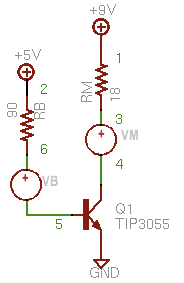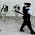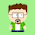## Tuesday, June 23, 2009

### SPICE: Part 3

<< [Part 1 2 3 4 5] >>

Since the last article, part 2, I've been refreshing my memory on the topic of BJTs, cracking open my old college text, Microelectronic Circuits (Sedra, Smith), which I recommend as a good reference. There's a newer, way more expensive edition available (Microelectronic Circuits Revised Edition) but I think the 2nd edition is good enough.

At any rate, I wanted to start from scratch with the motor, and work outward from there to try and come up with a better circuit.

The Motor

For now, I'll continue using a basic resistor which is probably good enough for steady state (operating point) analysis. I found a facsimilie of the motor I'm using. The Mabuchi FK-180SH-14800 is a 12V motor of approximately the same size that uses 0.34A at maximum efficiency. Let's say my motor uses 0.5A at 9V. In which case the motor's steady state resistance would be about 18Ω. So how do we use the TIP3055 to run this motor? (Yes, I realize that using a 15A transistor to drive a 0.5A load is overkill... it was just what I had on hand at the time)

Transistor Saturation and Topology

We want to drive the TIP3055, an NPN Bipolar Junction Transistor (BJT), into saturation. In this state, collector current, IC, is essentially at a maximum despite any additional base current, IB, that we will want to add. As you might expect, then, BJTs enter saturation mode when

IB > IC ÷ ß

Typically one wants to drive the transistor well into saturation by making sure IB is 2-10 times the saturation value of IB. This is called the overdrive factor. Ultimately, we control the amount of base current by selecting the an appropriately sized base resistor, RB.

Note that if we stick the motor on the transistor's emitter, then it limits how much extra base current we can supply at the base. Instead, it is typical practice to place the resistive load of the motor bewteen the voltage source and the collector terminal. That way the only resistance limiting IB is the base resistor.Selecting the Base Resistor

Our first step is to determine the saturation collector current, ICsat. To do this, we analyze the circuit (right) using Kirchoff's Voltage Law where we set VBE=0: ICsat = (VCC - VCEsat) / RM ; then calculate IBsat = ICsat ÷ ß and finally, select our desired IB = 10•IBsat

The book says to assume VCEsat is approximately 0.3V. Upon looking at the data sheet for the TIP3055, actually varies depending on the IC but as long as we estimate VCEsat on the low side, it seems to me that we'd end up with a larger IB which will only ensure we are driving the transistor well into saturation.

The value of ß, as I understand it, is equal to hFE (DC current gain) for a given IC, as specified by the data sheet. Looking up an IC of 0.5A, hFE = 100. Working the numbers, we get

ICsat = (9 - 0.3) / 18 = 480mA
IBsat = 480mA ÷ 100 = 4.8mA

And with an overdrive factor of 10,

IB = 10•4.8mA = 48mA

Now that we know what IB we're looking for, we simply solve for RB = (VCC - VBE) ÷ IB. The book says to use VBE = 0.7V. We get:

RB = (9 - 0.7) ÷ 48mA = 173Ω

But I want to drive the circuit with a 5V signal from a microcontroller (the ATmega168) Hooking RB to a 5V source instead of 9V we get:RB = (5 - 0.7) ÷ 48mA = 90Ω

SPICE Model

So let's whip up a SPICE model and see if that matches up with what we've done so far. Here's the circuit (right) with the nodes labeled. We're using a 0V supply, VM, to measure IC and similarly the 0V supply, VB, measures IB. We'll continue to use the same TIP3055 model as before and we'll set up the resistors per calculations (RB=90Ω and RM=18Ω)

This time, let's do an operating point analysis. As mentioned previously, the OP command tells SPICE to find out the steady-state of the circuit and the PRINT commands specify what variables to print. We are interested in the base voltage, V(5); the collector-emitter voltage, V(3); and of course the base current, I(VB) and collector current, I(VM).

Below is the SPICE deck to accomplish all that.
Motor driverV9 1 0 9.0V5 2 0 5.0RM 1 3 18VM 3 4 0Q1 4 5 0 TIP3055VB 6 5 0RB 2 6 90.MODEL TIP3055 NPN(Is=457.5f Xti=3 Eg=1.11 Vaf=50+ Bf=156.7 Ise=1.346p Ne=1.34+ Ikf=3.296 Xtb=2.2 Br=7.639 Isc=604.1f Nc=2.168+ Ikr=8.131m Rc=91.29m Cjc=278.7p Mjc=.385 Vjc=.75+ Fc=.5 Cje=433p Mje=.5 Vje=.75 Tr=1.412u Tf=37.34n+ Itf=35.68 Xtf=1.163 Vtf=10 Rb=.1).controlopprint v(4) i(vm) v(5) i(vb).endc.end
And when we run it, we get numbers that actually kind of match what we expected. Cool. Our IC is about 490 mA, IB is 47 mA and VBE is about 0.77 V. It's not exact because we were using some estimates, but it's close enough.
MacSpice 45 -> source Macintosh\ HD:Desktop:ex3.cirCircuit: Motor driverv(4) = 1.177197e-01i(vm) = 4.934600e-01v(5) = 7.675994e-01i(vb) = 4.702667e-02
Note that the ratio of IC to IB is 10, considerably less than a ß (hFE) of 100 that we'd expect in active mode for a collector current of about 0.5A. In other words, we're forcing in extra base current without any appreciable gain in collector current. That's saturation.

Incidentally, if you want to see what happens when the 5V signal is switched off, just revise the deck to tie RB to ground. Here's what happens to the voltages and currents:
v(4) = 9.000000e+00i(vm) = 2.201261e-11v(5) = 9.757939e-10i(vb) = -1.08422e-11
Base and collector current are negligible and there's no voltage drop across RM or RB. In a word, the transistor is off. Just what we expected.

Too Much Signal Current

One little gotcha. As I mentioned, I want to drive this circuit with an ATmega168 which is limited to 40mA per data pin, according to the datasheet. So in the next article I'll incorporate a second transistor to drive the first and add that to the SPICE model above and see what we can come up with.

<< [Part 1 2 3 4 5] >>

1.2.